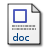# SISTEM PERSAMAAN LINEAR FUZZY

Noranita, Beta (2008) SISTEM PERSAMAAN LINEAR FUZZY. Jurnal Matematika dan Komputer, 11 (2). pp. 94-99. ISSN 1410-8518Microsoft Word - Published Version338Kb

## Abstract

Let AU = V be fuzzy system of linear equations. The fuzzy system of linear equations consist of n variables and n equations.We rearrange the fuzzy system of linear equations became 2n variables and 2n equations. The new system was devoted by BX* = V*. In this paper, we show that solution of BX* = V* can be used to find AU = V fuzzy system of linear equations, whenever B-1 is non-negative.

Item Type: Article Fuzzy numbers, system of linear equations, and non-negative matrix. Q Science > QA Mathematics > QA75 Electronic computers. Computer science Faculty of Science and Mathematics > Department of Computer Science 1950 Admin Departemen Matematika 01 Dec 2009 01:12 01 Dec 2009 01:12

Repository Staff Only: item control page